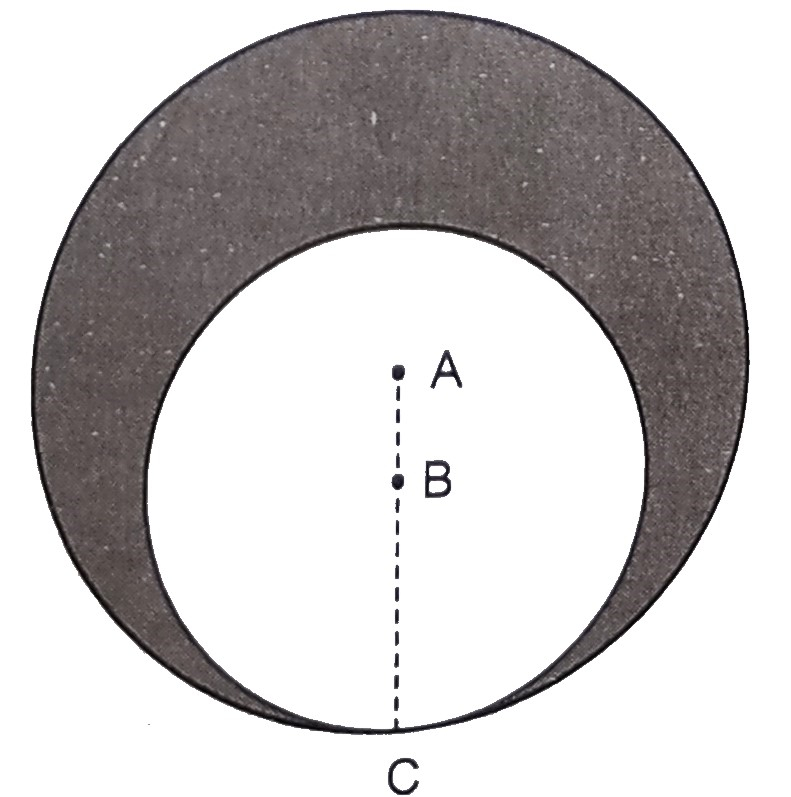"
">

# In the figure below, two circles with centres $A$ and $B$ touch each other at the point $C$. If $A C=8 \mathrm{~cm}$ and $A B=3 \mathrm{~cm}$, find the area of the shaded region."

Given:

Two circles with centres $A$ and $B$ touch each other at the point $C$.

$A C=8 \mathrm{~cm}$ and $A B=3 \mathrm{~cm}$.

To do:

We have to find the area of the shaded region.

Solution:

$BC = 8 - 3\ cm$

$= 5\ cm$

Radius of the bigger circle $R= 8\ cm$

Radius of the smaller circle $r = 5\ cm$

Therefore,

Area of the shaded region $=$ Area of the bigger circle $-$ Area of the smaller circle

$=\pi R^{2}-\pi r^{2}$

$=\frac{22}{7}(8^{2}-5^{2})$

$=\frac{22}{7}(64-25)$

$=\frac{22}{7} (39)$

$=122.57 \mathrm{~cm}^{2}$

The area of the shaded region is $122.57\ cm^2$.

Updated on: 10-Oct-2022

167 Views